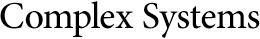Evaluating the Complexity of Mathematical Problems: Part 2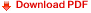Cristian S. Calude
University of Auckland, New Zealand
www.cs.auckland.ac.nz/~cristian

Elena Calude
Massey University at Albany, New Zealand
www.massey.ac.nz/~ecalude

Abstract

In this paper we present an implementation of the computational method in  that allows ranking mathematical statements by their complexity. We introduce the complexity classes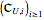, and, accordingly, show that Legendre's conjecture, Fermat's last theorem, and Goldbach's conjecture are in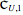, Dyson's conjecture is in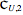, the Riemann hypothesis is in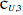, and the four color theorem is in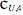.